MATH 480:

Homework # 3

1.Using WCS theorem find the optimal solution to the following L.P.Ps.

a. max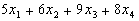s. t.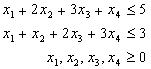b. max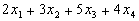s.t.c. min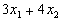s.t.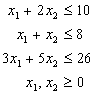2. Given the L.P.P

mins.t.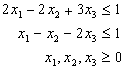the final tableau for this program (verify) is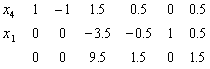add newwith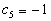and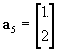Using the above tableau find the new optimal solution.